### Home > MC2 > Chapter Ch7 > Lesson 7.1.1 > Problem7-11

7-11.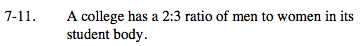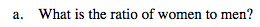If there are 2 men for every 3 women, what would the ratio of women to men be?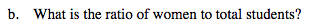If for every 3 women there are 2 men, the total students per 3 women is 5. What is this ratio?

3:5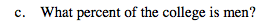If the ratio of men to total students is 2:5, what percentage would this represent?

40%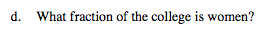Based on the ratio of women to total students from part (b), what would the fraction for women out of total students be?